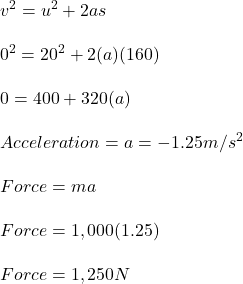Question

A 1000-kg car is driving toward the north along a straight horizontal road at a speed of 20.0 m/s. The driver applies the brakes and the car comes to a rest uniformly in a distance of 160 m. What are the magnitude and direction of the net force applied to the car to bring it to rest?

1.huyenthanh

Force applied to stop the car = 1,250 N

Explanation:

Given:

Mass of car (M) = 1,000 kg

Initial velocity (U) = 20 m/s

Final velocity (V) = 0 m/s

Distance (S) = 160 m

Find:

Force applied to stop the car.

Computation:Force applied to stop the car = 1,250 N